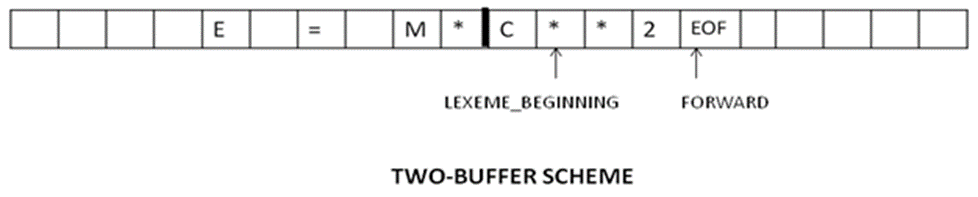Question:
Published on: 1 October, 2023

What do you mean by input buffering? How is input buffering implemented? What problem can arise implementing input buffering? Give suitable example. What is sentinel? What is its use?

The Lexical Analyzer scans the characters of the source program one at a time to discover tokens. Because of large amount of time can be consumed scanning characters, specialized buffering techniques have been developed to reduce the amount of overhead required to process an input character.

Buffering techniques:

1. Buffer pairs
2. Sentinels

Often, however, many characters beyond the next token many have to be examined before the next token itself can be determined. For this and other reasons, it is desirable for the lexical analyzer to read its input from an input buffer. Hence we introduce a two-buffer scheme that handles large lookaheads safely. We then consider an improvement involving "sentinels" that saves time checking for the ends of buffers.

Buffer Pairs:

Each buffer is of the same size N, and N is usually the size of a disk block, e.g., 4096 bytes.

• Using one system read command we can read N characters into a buffer.
• If fewer than N characters remain in the input file, then a special character, represented by eof, marks the end of the source file.
•Two pointers to the input are maintained:
• Pointer lexeme_beginning, marks the beginning of the current lexeme, whose extent we are attempting to determine.
• Pointer forward scans ahead until a pattern match is found.
• Once the next lexeme is determined, forward is set to the character at its right end.
• Then, after the lexeme is recorded as an attribute value of a token returned to the parser, lexeme_beginning is set to the character immediately after the lexeme just found.
• Advancing forward requires that we first test whether we have reached the end of one of the buffers, and if so, we must reload the other buffer from the input, and move forward to the beginning of the newly loaded buffer.
if forward at end of first half then begin
forward := forward + 1
end
else if forward at end of second half then begin
move forward to beginning of first half
end
else
forward := forward + 1;
end


Problem implementing input buffering:

Advancing forward requires that we first test whether we have reached the end of one of the buffers, and if so, we must reload the other buffer from the input, and move forward to the beginning of the newly loaded buffer. As long as we never need to look so far ahead of the actual lexeme that the sum of the lexeme's length plus the distance we look ahead is greater than N, we shall never overwrite the lexeme in its buffer before determining it.

For example, in a PL/I program we may see: DECALRE (ARG1, ARG2… ARG n) without knowing whether DECLARE is a keyword or an array name until we see the character that follows the right parenthesis. In either case, the token itself ends at the second E. If the lookahead pointer travels beyond the buffer half in which it began, the other half must be loaded with the next characters from the source file. Since the buffer shown in above figure is of limited size there is an implied constraint on how much look ahead can be used before the next token is discovered. In the above example, if the look ahead traveled to the left half and all the way through the left half to the middle, we could not reload the right half, because we would lose characters that had not yet been grouped into tokens. While we can make the buffer larger if we chose or use another buffering scheme, we cannot ignore the fact that overhead is limited.

Sentinels:

• The sentinel is a special character that cannot be part of the source program, and a natural choice is the character eof.
• Note that eof retains its use as a marker for the end of the entire input.
• Any eof that appears other than at the end of a buffer means that the input is at an end.
forward : = forward + 1;
if forward ↑ = eof then begin
if forward at end of first half then begin
forward := forward + 1
end
else if forward at end of second half then begin
move forward to beginning of first half
end
else /* eof within a buffer signifying end of input */
terminate lexical analysis
end


In the previous scheme, we must check each time the move forward pointer that has not moved off one half of the buffer. If it is done, then must reload the other half.

–     Therefore the ends of the buffer halves require two tests for each advance of the forward pointer.

–     This can reduce the two tests to one if it is extend each buffer half to hold a sentinel character at the end.

Random questions